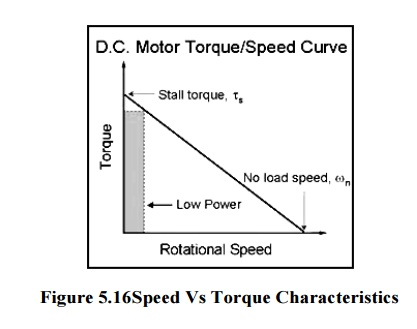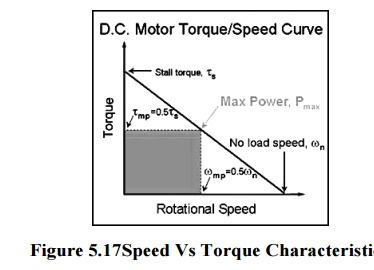Home | | Electrical Machines I | DC Motor Characteristics

# DC Motor Characteristics

1. Torque/Speed Curves 2. Power/Torque And Power/Speed Curves

Motor Characteristics

## 1. Torque/Speed Curves

In order to effectively design with D.C. motors, it is necessary to understand their characteristic curves. For every motor, there is a specific Torque/Speed curve and Power curve.The graph above shows a torque/speed curve of a typical D.C. motor. Note that torque is inversely proportional to the speed of the output shaft. In other words, there is a tradeoff between how much torque a motor delivers, and how fast the output shaft spins. Motor characteristics are frequently given as two points on this graph:

Â·                 The stall torque represents the point on the graph at which the torque is a maximum, but the shaft is not rotating.

Â·                 The no load speed, is the maximum output speed of the motor (when no torque is applied to the output shaft).

Â·                 The linear model of a D.C. motor torque/speed curve is a very good approximation. The torque/speed curves shown below are actual curves for the green maxon motor (pictured at right) used by students in 2.007. One is a plot of empirical data, and the other was plotted mechanically using a device developed at MIT.Note that the characteristic torque/speed curve for this motor is quite linear. This is generally true as long as the curve represents the direct output of the motor, or a simple gear reduced output. If the specifications are given as two points, it is safe to assume a linear curve.Recall that earlier we defined power as the product of torque and angular velocity. This corresponds to the area of a rectangle under the torque/speed curve with one corner attheorigin and another corner at a point on the curve. Due to the linear inverse relationship between torque and speed, the maximum power occurs at the point where Recall that earlier we defined power as the product of torque and angular velocity.This corresponds to the area of a rectangle under the torque/speed curve with one corner at the origin and another corner at a point on (t w), and  = Â˝ .## 2. Power/Torque And Power/Speed CurvesStudy Material, Lecturing Notes, Assignment, Reference, Wiki description explanation, brief detail
Electrical machines : DC Motor : DC Motor Characteristics |